Question

Consider the following chemical reaction: Cl2 (g) + 2 NaBr (s) ––> 2 NaCl (s) + Br2 (l)

What substance is oxidized in this reaction? (Hint: The substance that is oxidized will contain an element that undergoes an increase in oxidation number during the course of the reaction.)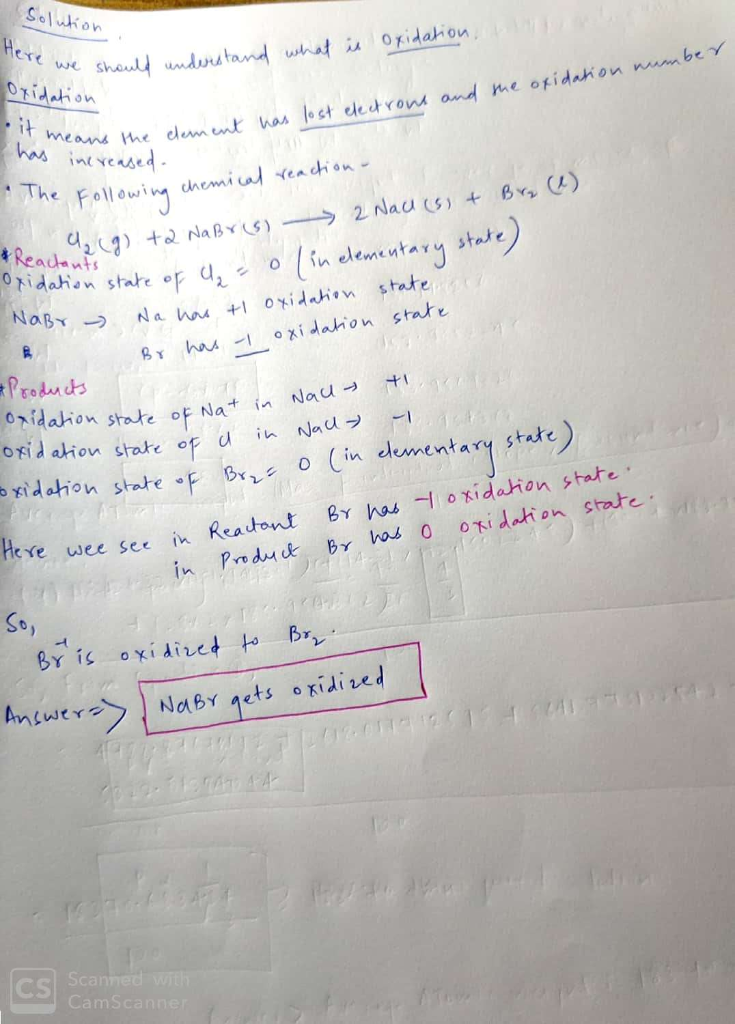#### Earn Coins

Coins can be redeemed for fabulous gifts.

Similar Homework Help Questions
• ### What is the coefficient for NaBr in the balanced version of the following chemical equation: Cl2+NaBr→NaCl+Br2

What is the coefficient for NaBr in the balanced version of the following chemical equation: Cl2+NaBr→NaCl+Br2

• ### Match Each reaction to its reaction type: 2 NaBr + Cl2 → 2 NaCl + Br2...

Match Each reaction to its reaction type: 2 NaBr + Cl2 → 2 NaCl + Br2 Na2SO4 + CaCl2 → CaSO4 + 2 NaCl CH4 + 2 O2 → CO2 + 2 H2O CaCO3 → CaO + CO2 MgO + H2O → Mg(OH)2 HCl + NaOH → NaCl + H2O A. Decomposition B. Double Replacement C. Combustion D. Acid/Base E. Explosion F. Combination G. Single Replacement

• ### Consider the following UNBALANCED equation: Na(s) + Cl2(g) -> NaCl(s). a.) Calculate the limiting reagent when...

Consider the following UNBALANCED equation: Na(s) + Cl2(g) -> NaCl(s). a.) Calculate the limiting reagent when 55.0 g of react with 67.2 g of Cl2. (Show all work, and clearly state which is the limiting reagent.) b.) What is the theoretical yield, in grams, of NaCl? c.) If 105g of NaCl is produced from the reaction, what is the percent yield of NaCl?

• ### Consider the following chemical reaction: CH4 (g) + Cl2(g) + CCl4 (1) + CCl4 (I) +...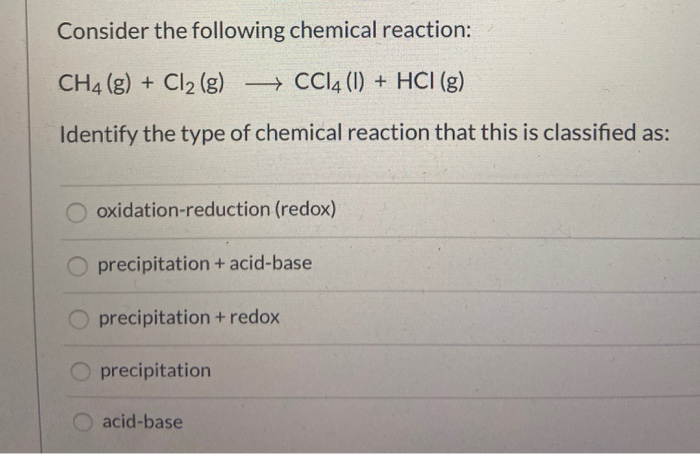Consider the following chemical reaction: CH4 (g) + Cl2(g) + CCl4 (1) + CCl4 (I) + HCl (g) Identify the type of chemical reaction that this is classified as: oxidation-reduction (redox) O precipitation + acid-base precipitation + redox precipitation acid-base

• ### Fill in the Blanks Consider the already balanced chemical reaction shown below: KCLO2(s)KCl(s) + O2(g) The...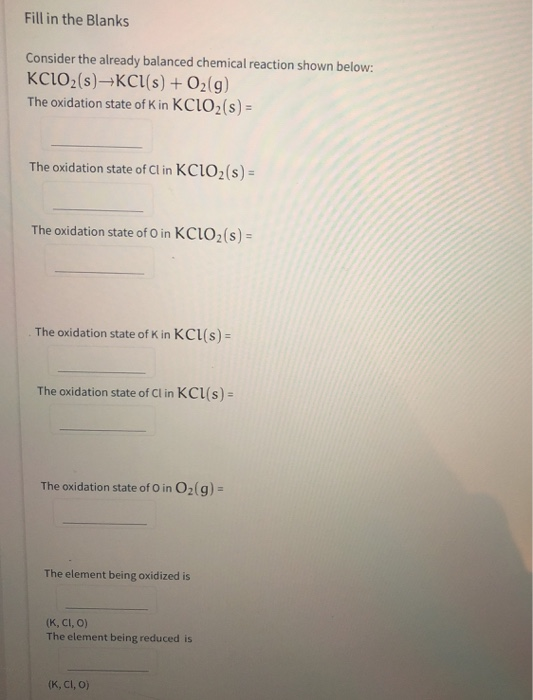Fill in the Blanks Consider the already balanced chemical reaction shown below: KCLO2(s)KCl(s) + O2(g) The oxidation state of K in KCLO2(s) = The oxidation state of Cl in KCLO2(s) = The oxidation state of O in KCLO2(s) = The oxidation state of K in KCl (s) = The oxidation state of Cl in Kcl(s) = The oxidation state of O in O2(g) = The element being oxidized is (K, CI, O) The element being reduced is (K, CI, O)

• ### Consider the following reaction, 2AB (g) ⇌ A2 (g) + B2 (g) K = 1.50x10−2 At...

Consider the following reaction, 2AB (g) ⇌ A2 (g) + B2 (g) K = 1.50x10−2 At some point in the reaction, Q = 5.30. Which of the following statements is correct concerning the equilibrium? 0.60 mol of Br2 and 0.60 mol of Cl2 are placed in a 1.00 L flask and allowed to reach equilibrium. After reaching equilibrium, the flask is found to contain 0.28 mol of BrCl. What is the value of Kc for this reaction? Br2  (g) + Cl2  (g)...

• ### Consider the following reaction: 2Na + Cl2(g) → 2NaCl

Consider the following reaction:2Na + Cl2(g) → 2NaCl ΔH = -821.8 kJ(a) Is the reaction exothermic or endothermic? (b) Calculate the amount of heat transferred when 5.6 g of Na reacts at constant pressure. (c) How many grams of NaCl are produced during an enthalpy change of 16.5 kJ? (d) How many kilojoules of heat are absorbed when 44.1 g of NaCl is decomposed into Na and Cl2 at constant pressure?

• ### A chemist measures the enthalpy change ΔH during the following reaction: 2Na (s) +   Cl2 (g)...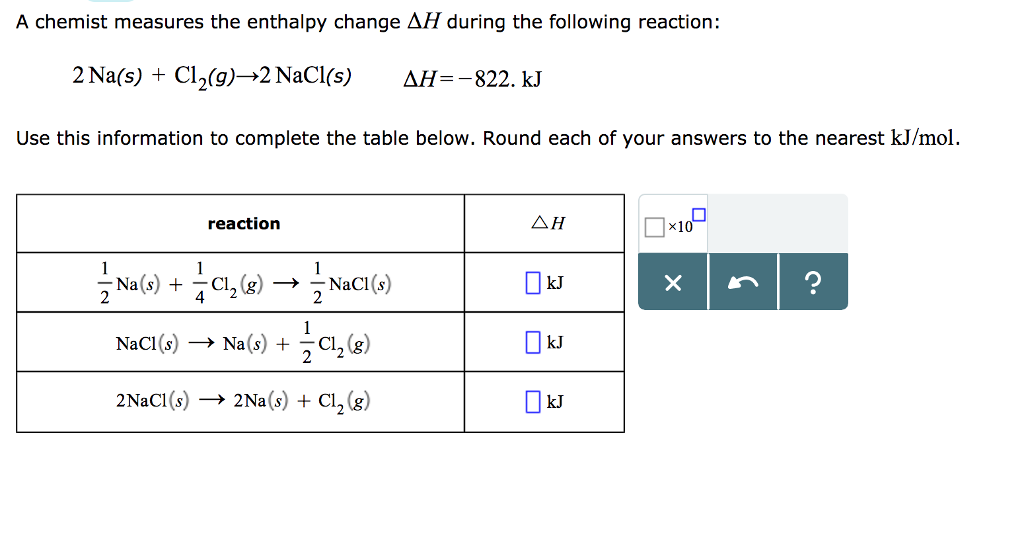A chemist measures the enthalpy change ΔH during the following reaction: 2Na (s) +   Cl2 (g) → 2NaCl (s) =ΔH−822.kJ Use this information to complete the table below. Round each of your answers to the nearest /kJmol . A chemist measures the enthalpy change AH during the following reaction: 2Na(s) + Cl2(g)→2 NaCl(s) NH=-822. kJ Use this information to complete the table below. Round each of your answers to the nearest kJ/mol reaction △H kJ NaCl(s) → Na(s) + Cl2(g)...

• ### Consider the balanced chemical reaction: Naz S (s)+2 HCI (aq) 2 NaCl (aq) + H,S (g)...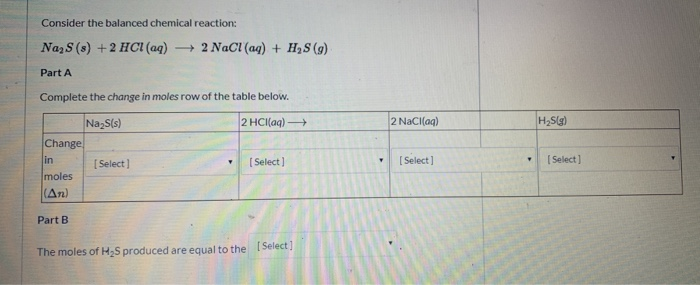Consider the balanced chemical reaction: Naz S (s)+2 HCI (aq) 2 NaCl (aq) + H,S (g) Part A Complete the change in moles row of the table below. H2Slg) 2 NaCl(aq) Na2S(s) Change 2 HCl(aq) [Select ] in [Select] [Select [Select] moles (An) Part B [Select The moles of H2S produced are equal to the 2 NaCl (aq) + H2 Na2S (s) +2 HCl (aq) Part A Complete the change in moles row of the table below. 2 HCl(aq) Na2S(s)...

• ### 3. Consider the following UNBALANCED equation: Na (s) Cl2(g) NaCl (s) + → a. Calculate the...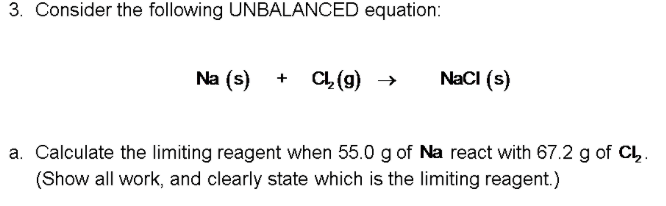3. Consider the following UNBALANCED equation: Na (s) Cl2(g) NaCl (s) + → a. Calculate the limiting reagent when 55.0 g of Na react with 67.2 g of CL, (Show all work, and clearly state which is the limiting reagent.)